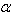SEARCH HOMEMath Central Quandaries & QueriesQuestion from Katrina, a student: I have already tried to do this problem but im having a very had time with it. Can you please help me. Glamour Magazine sponsored a survey of 2500 prospective brides and found that 60% of them spent less than $750 on their wedding gown. Use a 0.01 significance level to test the claim that less than 62% of brides spend less than$750 on their wedding gown. How are the results affected if it is learned that the responses were obtained from magazine readers who decided to respond to the survey through an Internet Web site?Hi Katrina,

This is a question about proportions so I know I will have to use the binomial distribution or the normal approximation to the binomial. It is a hypothesis test question about the parameter p so I read through the question carefully to see what I am asked to test. What I saw was

... test the claim that less than 62% ...

Thus is p is the population proportion I am to

test the claim that p < 0.62

Since this statement does not contain the equal sign it can not be the null hypothesis and the null hypothesis must be that this is not true, that is that p ≥ 0.62. Besides that I see that this is a one sided test. Now I can see what to do.

Let p be the proportion of brides who spend less than $750 on their wedding gown. A sample of 2500 prospective brides found that 60% of them spent less than$7500 on their wedding gowns. Thus n = 2500. The hypotheses test is then

H0 : p ≥ 0.62
H1 : p < 0.62= 0.01

Can you complete it now?
PennyMath Central is supported by the University of Regina and The Pacific Institute for the Mathematical Sciences.